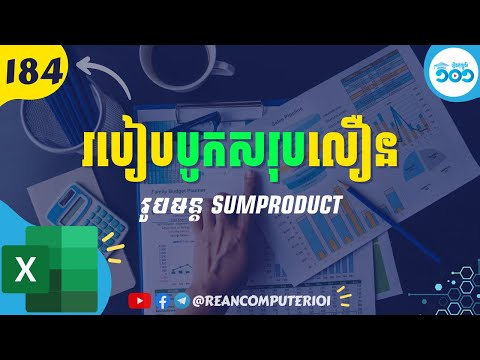# How to SUMPRODUCT formula in Excel with Example Download and Video demo

In this tutorial show two main formula, PRODUCT and SUMPRODUCT formula in EXCEL. SUMPRODUCT is the quick way to summary sale data without calculate sub total and then sum all together. You will able to know the different between SUMPRODUCT and PRODUCT and SUM in Excel.⚡ How to Download and Install Khmer Unicode for Windows and Mac OSX (apple)

## SUMPRODUCT formula in Excel

SUMPRODUCT returns the sum of products of ranges or arrays.
FORMULA:
=SUMPRODUCT(array1, [array2], [array3], ...)
◾ array1: Required, first array you want to multiply then add together.
◾ [array2],[array3],...: optional, array arguments from 2 to 255 to multiply then add.

## PRODUCT formula in Excel

PRODUCT returns the multiply all the numbers of arguments.
FORMULA:
=PRODUCT(number1, [number2], ...)
◾ number1: Required, first number to multiply.
◾ [number2],[number3],...: optional, additional numbers you want to multiply, up to 255 arguments.

Watch the video for more detail in Khmer